# Sumif MS Excel How To

## Step by Step: How to Use SumIF in excel 2003, 2007 & 2010 for Sum on a condition

Sumif MS Excel How To. How to sum column data where certain condition meets? Excel has Sum Function that sums up data cells where cells contain digit or numbers in the column on which this formula is applied. A user has to specify certain condition for this purpose. SUMIF is a great and useful formula for taking sum of values which meet certain criteria specified elsewhere.

### Step 1:

(b) Formulate your tables: these may be on the same worksheet or on a different worksheet and may even be on other workbooks.
(c) For the sake of example we have created two Tables in d and e:

(d) Table from where data is to be retrieved: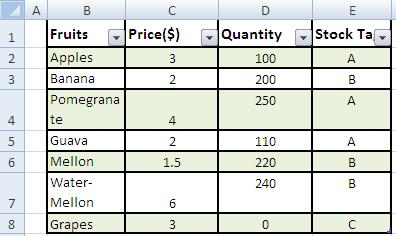Ms Excel Sumif Function

(e) Table where data is to be fetched/desired.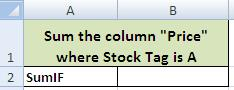Using Excel Sumif

### Step 2:

Ensure spellings for search values are the same in both the tables.

### Step 3:

Make sure there is no extra space.

### Step 4: Rechecking and reviewing the step by step final Sumif formula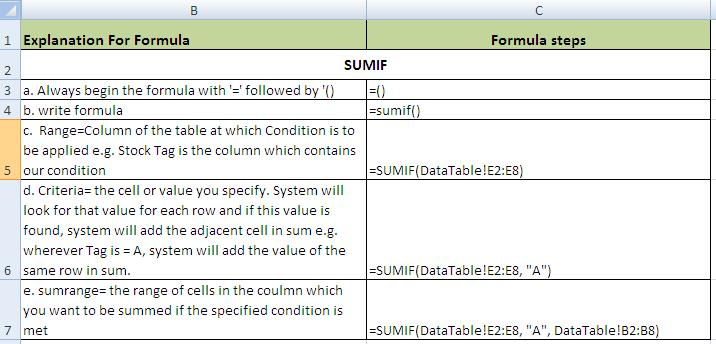Excel Sumif

### Final Formula of MS Excel for Sumif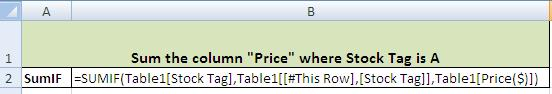Excel 2007 2010 sumif

### Result of SUMIf formula excel: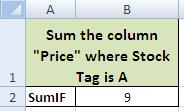Sumif in excel 2007